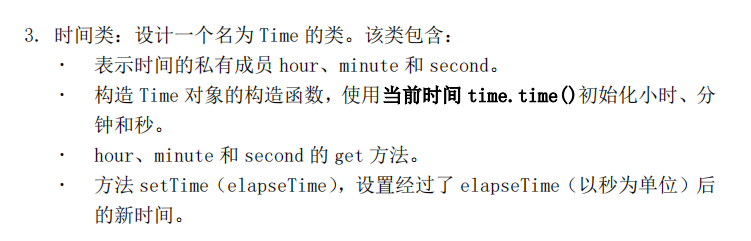2021-05-08 08:16

# Python 设计一个表示时间的类• 写回答
• 关注问题
• 收藏
• 邀请回答

#### 1条回答默认 最新

•kaili_ya 2021-05-08 09:08
已采纳
``````import time
class Time():
def __init__(self):
times = time.localtime(time.time())
self.__hour__ = times.tm_hour
self.__minute__ = times.tm_min
self.__second__ = times.tm_sec

def get_hour(self):
return self.__hour__
def get_minute(self):
return self.__minute__
def get_second(self):
return self.__second__

def setTime(self, elapseTime):
hour =  self.__hour__
minute =  self.__minute__
second = self.__second__

if ((hour >= 0) and (hour <24) ) and ((minute>= 0) and (minute <=60)) and ((second>= 0) and (second <=60)):
second += elapseTime
if second >= 60:
second = second-60
minute += 1

if minute >= 60:
minute = minute-60
hour += 1

if hour ==24:
hour = 0
return '%02d:%02d:%02d'%(hour,minute,second)

t = Time()
print(t.get_hour())
print(t.get_minute())
print(t.get_second())
print(t.setTime(50))``````
打赏 评论